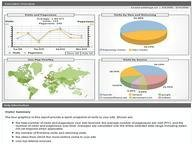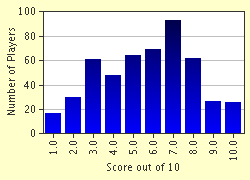FREE! Click here to Join FunTrivia. Thousands of games, quizzes, and lots more!# Practical Probability Trivia Quiz

### This is a basic probability quiz. It involves coin flips, dice rolls, spinning spinners, and other things. Just remember, if you get stuck, make a logical guess! All answers will be expressed as common fractions.

A multiple-choice quiz by xxharryxx. Estimated time: 5 mins.

Author
xxharryxx
Time
5 mins
Type
Multiple Choice
Quiz #
210,656
Updated
Aug 03 22
# Qns
10
Difficulty
Tough
Avg Score
5 / 10
Plays
2260
Last 3 plays: Guest 209 (4/10), ebanks120 (4/10), Guest 122 (8/10).
This quiz has 2 formats: you can play it as a or as shown below.
Scroll down to the bottom for the answer key.
1. On a regular six-sided die, what is the probability that you will roll an even number if you throw the die? Hint

1/3
1/6
5/6
1/2

#### NEXT>

2. Let's say you and your friend play a game that involves several coin flips. You flip three coins at the same time. If all three coins come up the same (all heads or all tails), your friend wins. Otherwise, you win. What is the probability that you win? Hint

1/4
3/8
3/4
1/2

#### NEXT>

3. A spinner is divided into five equal sections, with each section having a different number from 1-5 written on it. When you spin the spinner once, the arrow lands on 1. You spin the spinner a second time. What is the probability that it lands on 1 again? Hint

1/25
1/5
0
2/5

#### NEXT>

4. You play a game in which you flip a coin until you get tails. Each time your coin comes up heads, you win \$200. If you play the game only once, what is the probability that you make exactly \$1000? Hint

1/32
1/2
1/16
1/64

#### NEXT>

5. A spinner is divided into twelve equal sections and each section is then labeled with a different number from one to twelve. What is the probability of spinning a composite number if you spin the spinner once? Hint

1/2
7/12
5/12
1/3

#### NEXT>

6. The probability of picking a red marble from a bag of red, blue, and yellow marbles is 7/15. The probability of picking a yellow marble is 20%. There are 15 blue marbles in the bag. How many total marbles are there in the bag?

#### NEXT>

7. To win a game, I must do three things: Flip heads on a fair coin toss, roll a composite number on a number cube, and spin a 2 on a spinner with three equal areas, each labeled with a different number 1-3. What is the probability that I win the game? Hint

1/8
2/9
1/9
1/18

#### NEXT>

8. There are four white marbles in a bag and six silver ones. Two marbles are drawn at random, one after the other. The first one is NOT replaced. What is the probability of drawing two white marbles in a row in your next draw? Hint

4/15
4/25
2/15
1/5

#### NEXT>

9. Determine the probability that a randomly selected number between one and ten (including one and ten) is a factor of 24. Hint

1/4
1/2
3/5
1/3

#### NEXT>

10. A couple has two children. At least one of them is male. What is the probability that one child is female, assuming the probability of having either sex is equal? Hint

3/4
1/4
1/2
2/3

 (Optional) Create a Free FunTrivia ID to save the points you are about to earn:Select a User ID:Choose a Password:Your Email:

Quiz Answer Key and Fun Facts
1. On a regular six-sided die, what is the probability that you will roll an even number if you throw the die?

There are six numbers on a die: 1, 2, 3, 4, 5, and 6. There are three even numbers on the die: 2, 4, and 6. 3 out of six numbers are even. 3/6 reduces to 1/2.
2. Let's say you and your friend play a game that involves several coin flips. You flip three coins at the same time. If all three coins come up the same (all heads or all tails), your friend wins. Otherwise, you win. What is the probability that you win?

A quick way of going about this problem is determining the probability that one of your friend's outcomes will appear. The probability of multiple events happening is equal to the product of the probability of each single event. The probability of flipping heads is 1/2, so the probability of flipping three heads in a row would be (1/2 * 1/2 * 1/2), or 1/8.

The same thing occurs in the tails. (1/2 * 1/2 * 1/2) is equal to 1/8. The probability of your friend winning is 2/8, or 1/4. Subtract 1/4 from 1 to get 3/4, the probability of you winning.
3. A spinner is divided into five equal sections, with each section having a different number from 1-5 written on it. When you spin the spinner once, the arrow lands on 1. You spin the spinner a second time. What is the probability that it lands on 1 again?

Even though the spinner just landed on 1, it doesn't change the outcome of the next spin. There is only one way to spin a 1, and there are five spots for the spinner to land on. The probability is 1/5.
4. You play a game in which you flip a coin until you get tails. Each time your coin comes up heads, you win \$200. If you play the game only once, what is the probability that you make exactly \$1000?

You have to flip heads five times in a row to win \$1000. The probability of flipping heads once is 1/2, so the probability of flipping heads five times would be (1/2 * 1/2 * 1/2 * 1/2 * 1/2), or 1/32. However, the game is not over yet. In order to end the game, you must now flip tails. The probability of that is 1/2. 1/2 * 1/32 = 1/64.
5. A spinner is divided into twelve equal sections and each section is then labeled with a different number from one to twelve. What is the probability of spinning a composite number if you spin the spinner once?

A composite number is a number with more than two factors. We see that 4, 6, 8, 9, 10, and 12 are composite when we look at their factors. 6 out of the 12 numbers are composite. 6/12 simplifies to 1/2.
6. The probability of picking a red marble from a bag of red, blue, and yellow marbles is 7/15. The probability of picking a yellow marble is 20%. There are 15 blue marbles in the bag. How many total marbles are there in the bag?

15 blue marbles has to be a fraction of the total amount of marbles. It is the fraction that the red and yellow marbles do not take up. To find the fraction, first convert 20% to a fraction. 20% is equal to 1/5. 7/15 + 1/5 (or 3/15) is equal to 10/15 or 2/3.

The red and yellow marbles take up 2/3 of the bag, so we know that the blue marbles must take up the remaining third of the bag. You can now set up a formula using the variable x, where x equals the total number of marbles in the bag. 1/3 * x = 15. Multiply each side by 3 (or divide by 1/3) to get x = 45 marbles.
7. To win a game, I must do three things: Flip heads on a fair coin toss, roll a composite number on a number cube, and spin a 2 on a spinner with three equal areas, each labeled with a different number 1-3. What is the probability that I win the game?

The probability of flipping heads is 1/2. The probability of rolling a composite number (4 and 6) is 1/3. The probability of spinning a 2 is 1/3. Multiply: 1/2 * 1/3 * 1/3 is equal to 1/18.
8. There are four white marbles in a bag and six silver ones. Two marbles are drawn at random, one after the other. The first one is NOT replaced. What is the probability of drawing two white marbles in a row in your next draw?

On the first draw, there are four white marbles out of ten. The probability of picking one is 2/5. On the second draw, assuming we picked a white marble, there are three white marbles out of nine total marbles. The probability of picking one is 1/3. Multiply the probabilities of both outcomes together to get 2/15.
9. Determine the probability that a randomly selected number between one and ten (including one and ten) is a factor of 24.

Looking at the factors of 24, we see that 1, 2, 3, 4, 6, and 8 are the factors of 24 that are between one and ten. Six out of the ten numbers between one and ten are factors of 24. 6/10 reduces to 3/5.
10. A couple has two children. At least one of them is male. What is the probability that one child is female, assuming the probability of having either sex is equal?

Since the male child could be the first or second child, the easiest way to see this problem would be to list out all possible outcomes. The couple could have a boy, then a boy. They could also have a boy and then a girl. They could have a girl first, and then a boy.

The final combination would be two girls. Since the problem states that at least one child is a boy, the final option of two girls does not work. Looking at our three remaining combinations, two of them have one female. The probability is 2/3.
Source: Author xxharryxx

This quiz was reviewed by FunTrivia editor crisw before going online.
Any errors found in FunTrivia content are routinely corrected through our feedback system.
Most Recent Scores
Jun 03 2023 : Guest 209: 4/10
Jun 02 2023 : ebanks120: 4/10
Jun 01 2023 : Guest 122: 8/10
Jun 01 2023 : Guest 198: 3/10
Jun 01 2023 : Guest 199: 3/10
May 30 2023 : Guest 174: 7/10
May 30 2023 : Guest 204: 7/10
May 30 2023 : Guest 185: 1/10
May 28 2023 : Guest 202: 2/10

Score Distribution